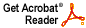# On the outputs of linear control systems

Joel H. Shapiro

J. Math. Anal. App. 340 (2008) 116--125

Abstract: This paper studies autonomous, single-input, single-output linear control systems on finite time intervals. The object of interest is the output operator, which associates to each input function and initial state vector the corresponding system output.

Main result: If the system has relative degree r, then for any "admissible" Banach space of inputs U, the output operator takes U x C^n boundedly onto the "Sobolev space" of complex-valued functions f in C^(r-1)([0, T ]) for which the r-th order derivative f^(r) is absolutely continuous, with f (r) in U.This completes recent results of Jonsson and Martin (J. Math. Anal. App. 329 (2007), 798--821) who showed that if the system is minimal and U is either L^2([0, T ]) or C([0, T ]), then the output operator is continuous, with range dense in U.

Download .pdf file (188KB)